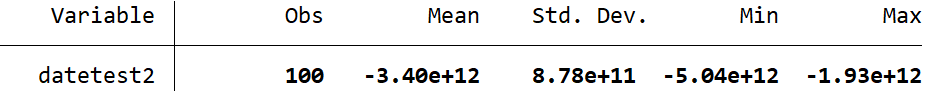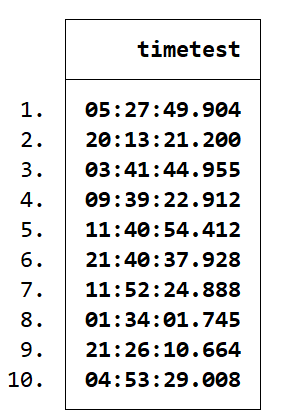Search

# How to Generate Random Test Variables

For complex analyses or new user-written commands it can be helpful to run tests using random variables. To create random variables in Stata, use the generate command with the runiform() or runiformint() functions. These functions will create a variable with randomised numbers.

If you are running tests that will need to be easily reproduced by others, you can save your test variables in a dataset for sharing. Alternatively, set the random seed before you create your test variables. As long as you set the seed the same and then generate the variables in the same order, you should get the same test variables.

## Worked Example 1 – a random variable with observations from 0 to 1

The runiform() function operates with the default range of 0 to 1. Let’s generate a random test variable. In the command pane:

This generates the variable testvar with the following characteristics:## Worked Example 2 – a random variable with float observations between 100 and 200

You can also use the runiform() function to specify a range within which to randomly generate numbers. For the runiform() function these numbers will be generated as floats rather than integers. We will cover generating random integers in the next example.

In the command pane:

This generates the variable testvar2 with the following characteristics:You will note I did not set the random seed for this variable. This is because it is not necessary to reset the seed for every random instance. If you set seed with the same number as shown in the first example, then set obs to 100 and generate testvar followed by testvar2, you should get the same results as I have here. As long as you perform the same commands in the same order once the random seed has been set, it will always be exactly the same.

## Worked Example 3 – a random variable with only integers between 100 and 200

To generate a random variable with only integers (i.e. no decimals) you use the runiformint() function. In the command pane:

This generates testvar3 with the following characteristics:## Worked Example 4 – a random date or date/time variable

You can use the runiformint() function with the td() or tc() functions to generate a random date or date/time variable. You will need to specify the start and end dates, however these can be any date at all. First I will generate a date-only variable:

This generates the following date variable:Now lets try generating a random date/time variable. In the command pane:

This gives the following characteristics to datetest2:And finally, to generate a random time-only variable, simply omit the date from the tc() function as follows:

This creates a random time variable with the following characteristics: